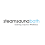## Tuesday, October 27, 2015

### Occam+Bayes=Induction

A classic problem in philosophy and the philosophy of science is how to justify induction. That is, how to rationally go from the fact that X is true in N previously observed cases to the belief that it is true in all cases, or at least in an additional, unobserved case. We will here propose a quick and simple method to justify induction, based on the combination of Occam's razor (to choose hypotheses) and Bayesian inference to update epistemic probabilities.

### Notation

Let us introduce the following notation. Let $H$ be some hypothesis which we want to judge for plausibility. Let $X_k$ be the fact that $X$ is true in the kth instance. Let $X^n$ be the fact that $X$ is true in the first n cases, that is $X^n=X_1 \cap X_2 \cap \cdots \cap X_n=\bigcap_{k=1}^{n}X_k$ so that $X^{n-1}\cap X_n=X^n$ Thus $P\left ( X^n|H \right )$ is the (epistemic) probability that we observe X in n cases, supposing H is true, and $P\left ( H|X^n \right )$ is the (epistemic) probability that H is true, supposing we observe X to be the case in n cases.

### Occam's Razor

There are three basic, simplest hypotheses we can form, all the rest being more complex. These three are the
• Proinductive (P) hypothesis: the chance of X happening again increases as we see more instances of it.
• Contrainductive (C) hypothesis: the chance of X happening again decreases as we see more instances of it.
• Uninductive (U) hypothesis: the chance of X happening again stays the same as we see more instances of it.
For concreteness, let $F_H(n)=P\left ( X_{n}|H \cap X^{n-1} \right )$. Thus we say that, for $m > 0$, $F_P(n+m) > F_P(n)$, and $\lim_{n \rightarrow \infty} F_P(n)=1$, and $F_C(n+m) < F_C(n)$, and $\lim_{n \rightarrow \infty} F_C(n)=0$, and $F_U(n)=F_U(0)$.

### Bayesian Inference

We want to find $P\left ( H|X^n \right )$ for the hypotheses listed in the previous section. We have $P\left ( X^n|H \right )=P\left ( X_n \cap X^{n-1}|H \right )=P\left ( X_n |X^{n-1} \cap H \right ) \cdot P\left ( X^{n-1} |H \right )=F_H(n) \cdot P\left ( X^{n-1} |H \right )$ Therefore $P\left ( X^n|H \right )=\prod_{k=1}^{n} F_H(k)$ Suppose that there are $N$ mutually exclusive and collectively exhaustive hypotheses. Then, Bayes' formula states: $P(H_m|A)=\frac{P(A|H_m)P(H_m)}{P(A|H_1)P(H_1)+P(A|H_2)P(H_2)+\cdots+P(A|H_N)P(H_N)}$ Thus, we have $P(H_m|X^n)=\frac{P(X^n|H_m)P(H_m)}{P(X^n|H_1)P(H_1)+P(X^n|H_2)P(H_2)+\cdots+P(X^n|H_N)P(H_N)}$ Therefore $P(H_m|X^n)=\frac{P(H_m)\prod_{k=1}^{n} F_{H_m}(k)}{P(H_1)\prod_{k=1}^{n} F_{H_1}(k)+P(H_2)\prod_{k=1}^{n} F_{H_2}(k) + \cdots + P(H_N)\prod_{k=1}^{n} F_{H_N}(k)}$ Let us suppose that the three hypotheses mentioned above are collectively exhaustive. Suppose, for concreteness that $F_P(n)=\frac{n}{n+1}$, $F_C(n)=\frac{1}{n+1}$, and $F_U(n)=\frac{1}{2}$. Thus $\prod_{k=1}^{n} F_{P}(k)=\frac{1}{n+1}$, and $\prod_{k=1}^{n} F_{C}(k)=\frac{1}{(n+1)!}$, and $\prod_{k=1}^{n} F_{U}(k)=\frac{1}{2^n}$. Let $P(P)=p$ and $P(C)=q$ and $P(U)=r$ where $p+q+r=1$. Then: $P(P|X^n)=\frac{p\frac{1}{n+1}}{p\frac{1}{n+1}+q\frac{1}{(n+1)!}+r\frac{1}{2^n}}$ $P(C|X^n)=\frac{q\frac{1}{(n+1)!}}{p\frac{1}{n+1}+q\frac{1}{(n+1)!}+r\frac{1}{2^n}}$ $P(U|X^n)=\frac{r\frac{1}{2^n}}{p\frac{1}{n+1}+q\frac{1}{(n+1)!}+r\frac{1}{2^n}}$ A simple assessment of limits shows that the former goes to 1 quite rapidly, for increasing n, for any nonzero p, and the latter two go to zero. In fact, for $p=q=r=1/3$, for $n>10$, $P(P|X^n)>0.99$, and for $n>17$, $P(P|X^n)>0.9999$.

This example is meant to be only illustrative, to show the general way in which Occam's razor, combined with Bayesian inference, leads to a support of induction. The same things happening repeatedly lends credence to the hypothesis that the same things happen repeatedly, and detracts from the hypothesis that the same things are unlikely to happen repeatedly, or always happen with the same probability. In a very similar way, a coin repeatedly coming up heads supports the hypothesis that it is biased to come up heads, and detracts from the hypotheses that it is biased to come up tails or is fair. This may seem obvious, but it is beneficial to see exactly how the mathematical machinery supports this intuition.

We may also wish to include other hypotheses, but we must first assess the prior probabilities that they are true, and Occam's razor advises taking the inverse probability as inverse to the complexity of the hypothesis. Thus, even if on the hypothesis, observing n X's is more likely than on the three discussed, it would needs be more complex or ad hoc, and so would have a significantly lower prior probability.

#### 1 comment:

1.Also, there are some acceptable ones out there as well! I'm simply more knowing with regards to where I invest my energy and cash now and I quit pursuing the following sparkly proposition." huum sauna heater.